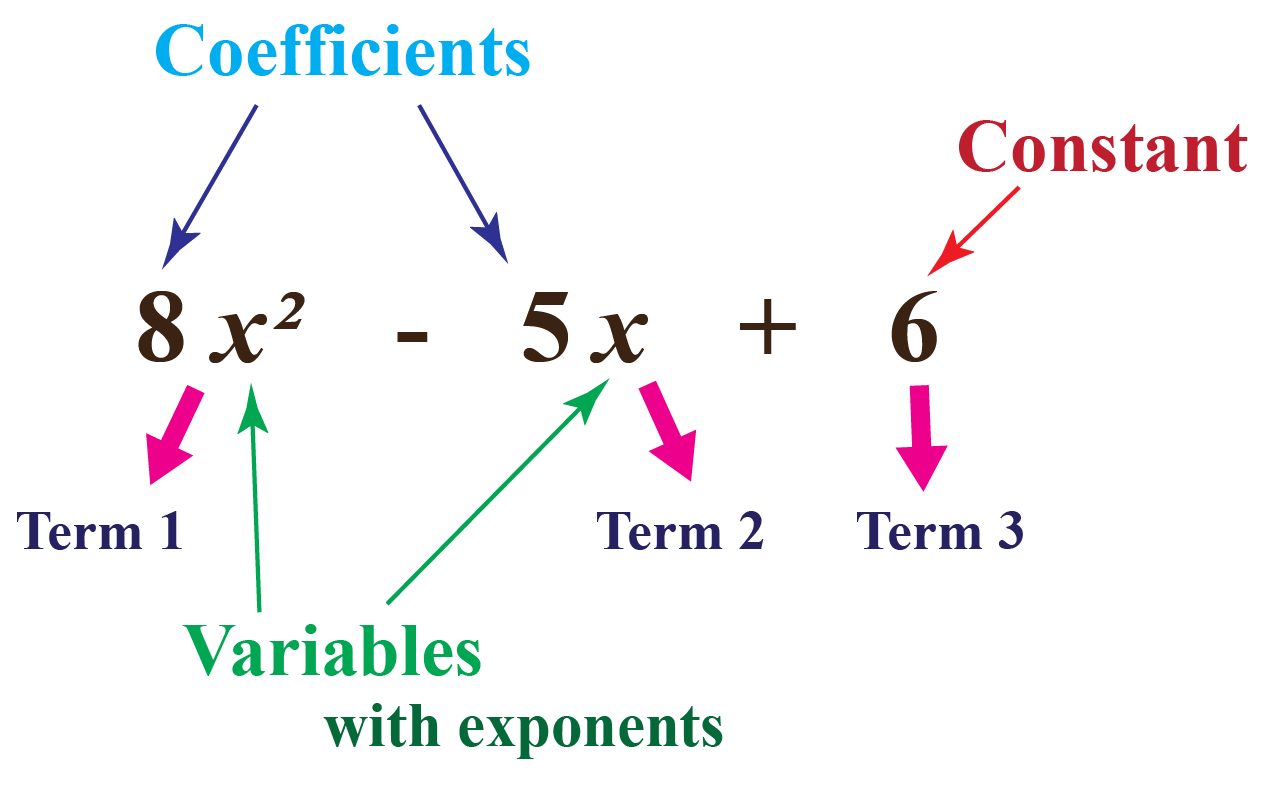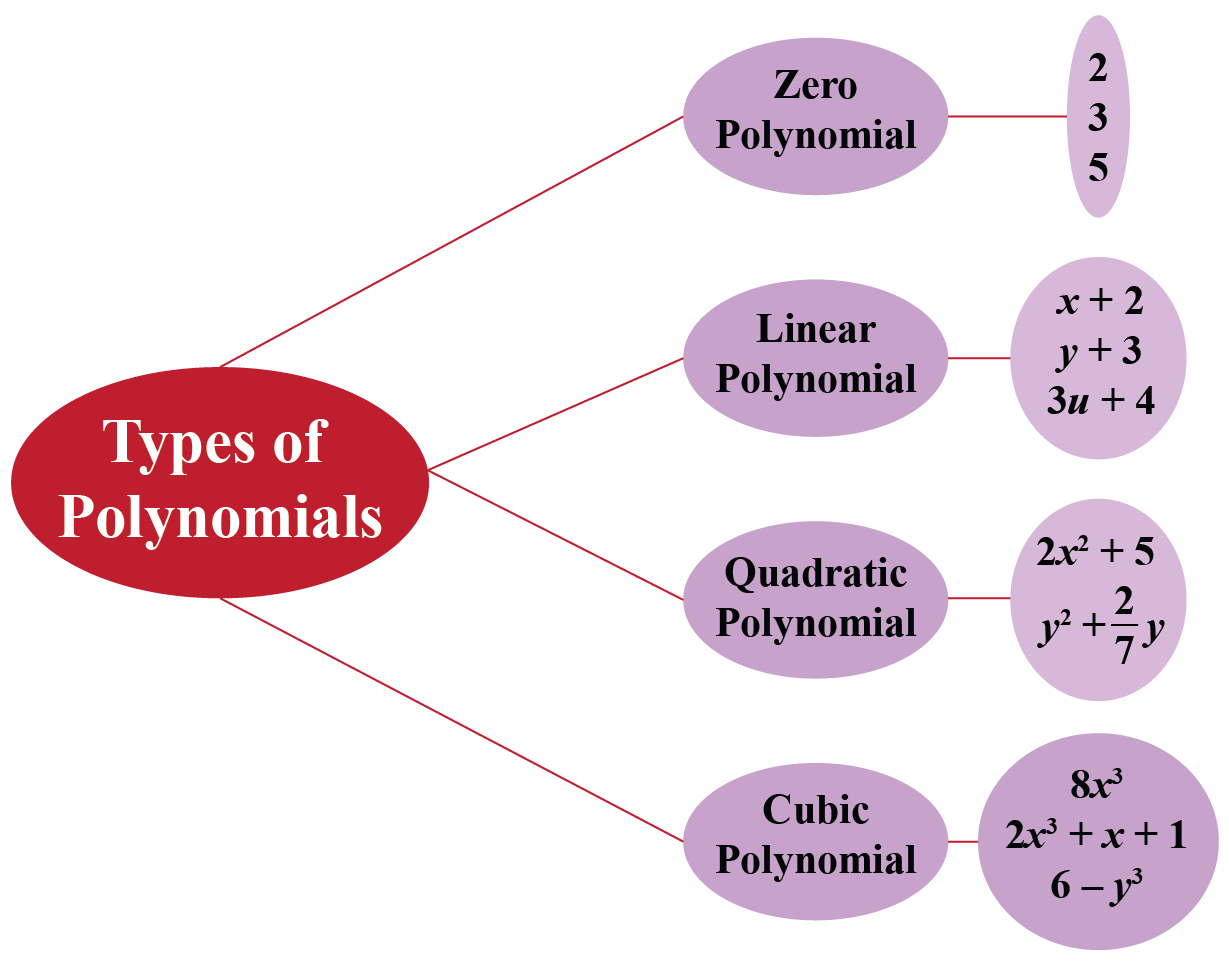# Polynomials in One Variable

Polynomials in One Variable

In algebra, it is very important to learn about polynomials.

Look at the basic form of a polynomial.There are many ways in which we can classify polynomials, and one such way is the number of variables present in the polynomial.

Can you tell how many variables are there in the above polynomial?

In this mini-lesson, we will learn about polynomials in one variable including its definition, classification based on degree, and polynomials in two variables through visualization, some solved examples, and a few interactive questions for you to test your understanding.

## Lesson Plan

 1 What Is the Definition of Polynomials in One Variable? 2 Tips and Tricks 3 Important Notes 4 Solved Examples 5 Interactive Questions

## What Is the Definition of Polynomials in One Variable?

In mathematics, a polynomial is an expression that consists of variables and coefficients, involving the operations of addition, subtraction, multiplication, and exponentiation of variables.

The word "polynomial" contains two words, namely, “poly" and “nomials”. "Poly" means many, and "nomials" means terms. Hence an expression containing many terms is called polynomials, having variables and coefficients.

Now, let's learn about the definition of polynomial in one variable.

Polynomials in one variable are those expressions in which there is only one variable present.

Some examples of polynomials in one variable are given below:

\begin{align} x^2+3x-2 \end{align}

\begin{align} 3y^3+2y^2-y+1 \end{align}

\begin{align} m^4-5m^2+8m-3 \end{align}Tips and Tricks
1. Before learning about polynomials in one variable, it is important to learn what do we consider as polynomials.
• Polynomials should have a whole number as the degree. Expressions with negative exponents are not polynomials. For example, $$x^{-2}$$ is not a polynomial.
• Polynomials do not have a variable in the denominator. For example, $$\dfrac{2}{x+2}$$ is not a polynomial.

## Classification of Polynomials in One Variable Based on Degree

To learn about the classification of one variable polynomial based on degree, let us first understand the meaning of the degree of a polynomial.

The highest power of the variable in a polynomial is called the degree of the polynomial. For example, in the following equation: $$x^2+2x+4$$, the degree of the polynomial is $$2$$, i.e., the highest power of the variable in the polynomial.

For a multivariable polynomial, it is the highest sum of powers of different variables in any of the terms in the expression.

Based on the degree of a polynomial in one variable, it can be classified into 4 types:

• Zero or constant polynomial
• Linear polynomial
• Quadratic polynomial
• Cubic polynomial### Zero or Constant Polynomial

A polynomial having 0 as the degree of the polynomial is termed as zero or constant polynomial. Such polynomials only have constant terms with no variable.

$$2$$, that we can also write as $$2x^0$$, is an example of zero polynomial.

### Linear Polynomial

A polynomial with 1 as the degree of the polynomial is termed as linear polynomial.

Linear polynomials can have multiple variables.

So, we consider linear polynomial in one variable as a type of polynomial in one variable.

These polynomials have only one solution.

Some examples are given below:

\begin{align} x+7 \end{align}

\begin{align} 3y-27 \end{align}

\begin{align} 7z+\dfrac{17}{2} \end{align}

### Quadratic Polynomial

A quadratic polynomial is a polynomial of degree 2, i.e, the highest exponent of the variable is 2

Quadratic polynomials in one variable have only two solutions possible.

Some examples are given below,

\begin{align} x^2+7x+12 \end{align}

\begin{align} 2m^2-5m+3 \end{align}

\begin{align} 8y^2-2 \end{align}

### Cubic Polynomial

A cubic polynomial is a polynomial of degree 3, i.e, the highest exponent of the variable is 3

Cubic polynomials in one variable have only three solutions possible.

Some examples are given below,

\begin{align} 4y^3-8 \end{align}

\begin{align} x^3+5x^2-6x-4 \end{align}

\begin{align} 5z^3-\dfrac{3}{4} \end{align}

## What Do You Mean by Polynomials in Two Variables?

Polynomials in two variables are expressions with two variables present in them. For example, $$x+y=5$$.

In polynomials in two variables, both the variables are dependent on each other.

Some examples of polynomials in two variables are given below.

\begin{align} x^3+y^3-xy=7 \end{align}

\begin{align} mn+m^2-8=0 \end{align}

\begin{align} 3p^5+q^4-32=0 \end{align}

\begin{align} a-3b=9 \end{align}

Like polynomials in one variable, polynomials in two variable can also be classified as linear polynomials, quadratic polynomials, and cubic polynomials.

Download Polynomials Worksheets
Polynomials
Grade 9 | Answers Set 1
Polynomials
Grade 10 | Questions Set 1
Polynomials
Grade 10 | Answers Set 1
Polynomials
Grade 9 | Questions Set 1
More Important Topics
Numbers
Algebra
Geometry
Measurement
Money
Data
Trigonometry
Calculus